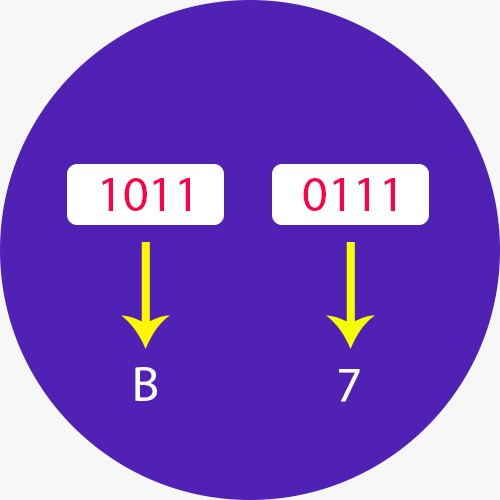﻿ Binary to Hex Converter | Binary to Hexadecimal Conversion Calculator

# Binary to Hex Converter

Use our binary to hex converter a free online calculator / conversion tool to convert binary value into hexadecimal number system.

#### Binary Number System

A Binary number system has only two digits that are 0 and 1. Every number (value) represents with 0 and 1 in this number system. The base of binary number system is 2, because it has only two digits.

A Hexadecimal number system has sixteen (16) alphanumeric values from 0 to 9 and A to F. Every number (value) represents with 0,1,2,3,4,5,6, 7,8,9,A,B,C,D,E and F in this number system. The base of hexadecimal number system is 16, because it has 16 alphanumeric values. Here A is 10, B is 11, C is 12, D is 14, E is 15 and F is 16.## How do you Convert Binary to Hexadecimal

An equally easy way to convert from binary to hexadecimal is to group binary digits into sets of four, starting with the least significant (rightmost) digits.

For Example: Convert the Binary Value: 11100010 to Hexadecial

Binary: 11100010 = 1110 0010

Then, look up each group in a table:Binary = 1110 0010

Hexadecimal = E 2 = E2 hex

0 0000 0 0
1 0001 1 1
2 0010 2 2
3 0011 3 3
4 0100 4 4
5 0101 5 5
6 0110 6 6
7 0111 7 7
8 1000 10 8
9 1001 11 9
10 1010 12 A
11 1011 13 B
12 1100 14 C
13 1101 15 D
14 1110 16 E
15 1111 17 F